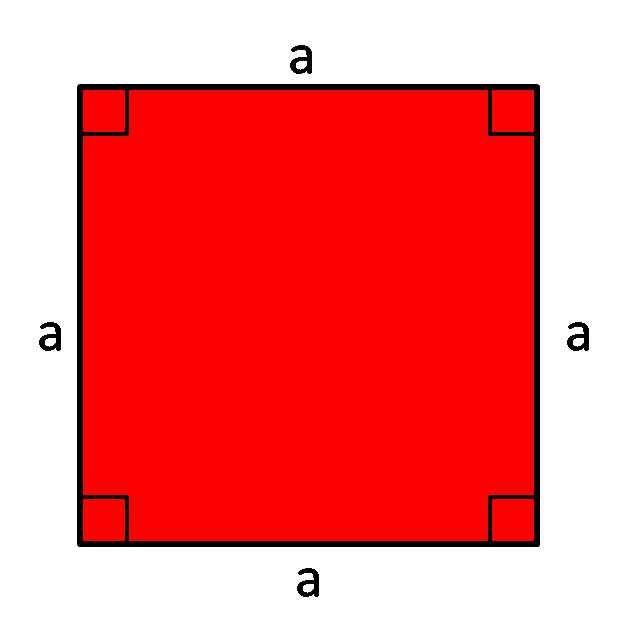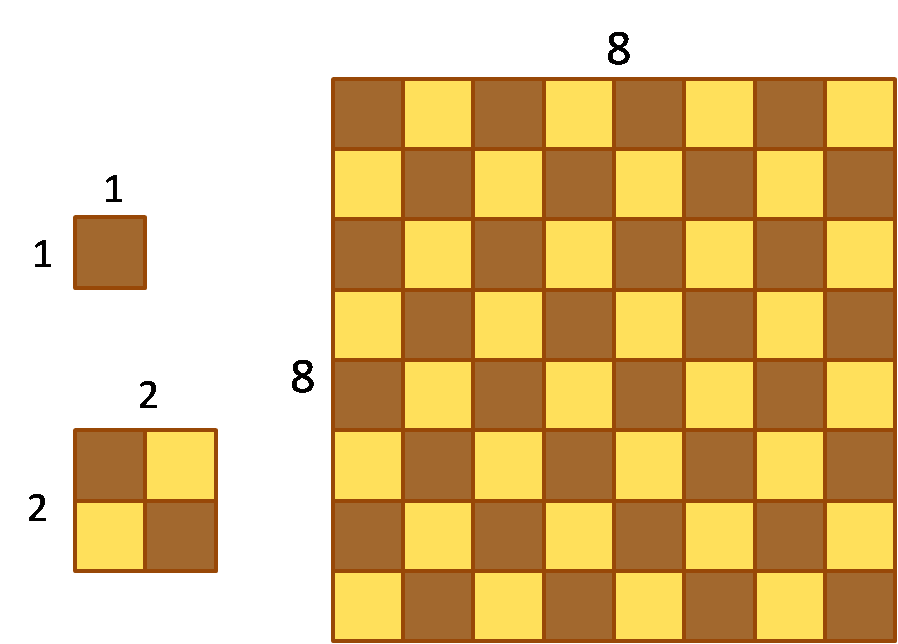# Area of a Square Calculator

Created by Hanna Pamuła, PhD candidate
Reviewed by Bogna Szyk
Last updated: Dec 15, 2021

If you forgot how to find the area of a square, you're in the right place - this simple area of a square calculator is the answer to your problems. Whether you want to find the area knowing the square side or you need to calculate the side from a given area, this tool lends a helping hand. Read on and refresh your memory to find out what is the area of a square and to learn the formula behind the calculator. If you also need to calculate the area and diagonal of a square, check out this square calculator.

## How to find the area of a square - formulasThe area of a square is the product of the length of its sides:

• area = a * a = a², where a is a square side

Other formulas also exist. Depending on which parameter is given, you can use the following equations:

• area = d² / 2 if you know the diagonal
• area = perimeter² / 16 if the perimeter is given
• area = 2 * R² knowing circumradius R
• area = 4 * r² in terms of the inradius r

## What is the area of a square?

The area of a square is a number of square units needed to completely fill a square. To understand that definition, let's have a look at this picture of a chessboard:The board has a squared shape, with its side divided into eight parts, in total it consists of 64 small squares. Assume that one small square has a side length equal to 1 in. The area of such square may be understood as the amount of paint necessary to cover the surface. So, from the formula for the area of a square, we know that area = a² = 1 in², and it's our unit of area in chessboard case (amount of paint). The area of 2x2 piece of the chessboard is then equal to 4 squares - so it's 4 in² and we need to use 4 times more "paint". Full chessboard area equals 84 in²: 8 in * 8 in from the formula or it's just 64 small squares with 1 in² area - so we need 64 times more "paint" than for one single square.

You may also be interested to check out the area of the largest square in a circle!

## How to use the area of a square calculator

Let's give the area of a square calculator a try!

1. Find out the given value. In our example, assume we know the side and we want to calculate the area.
2. Type it into proper box. Enter the value, e.g. 11 inches, into side box.
3. The area appears! It's 121 in². If you are interested how many square feet it is, change the unit by clicking on the unit name.
Hanna Pamuła, PhD candidateSide a
in
Area
in²
People also viewed…

### Car heat

The hot car calculator shows you how fast the interior of a car heats up during a summers day.

### Circumference of a cylinder

Use this circumference of a cylinder calculator to find a circumference of a cylinder.

### Oblique triangle

Calculate the area of a triangle in every possible way with the oblique triangle calculator!

### Pizza size

This calculator will help you make the most delicious choice when ordering pizza.